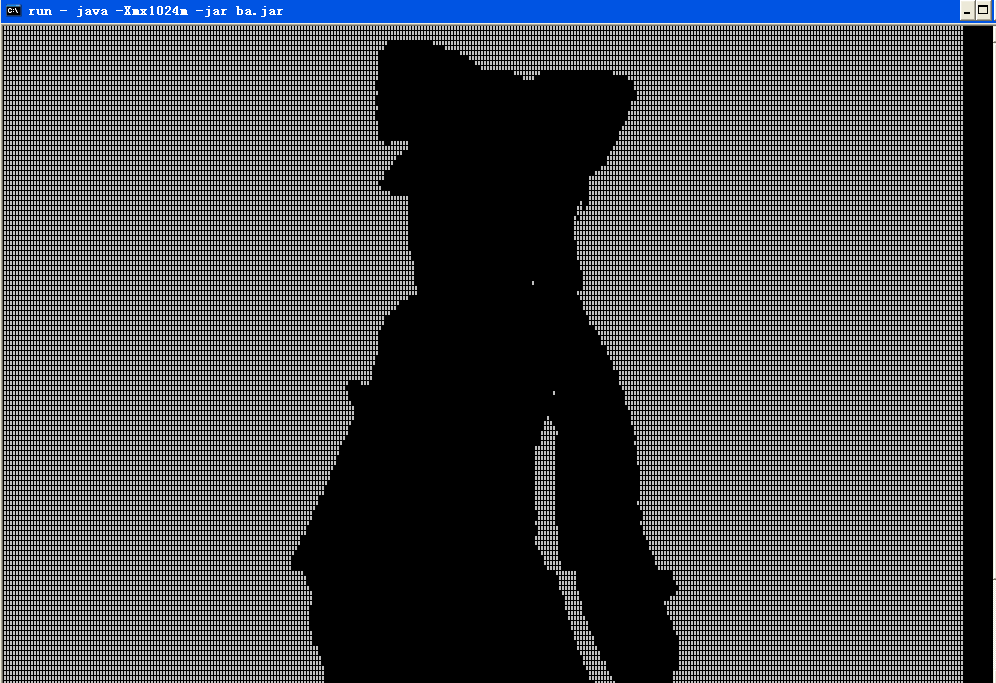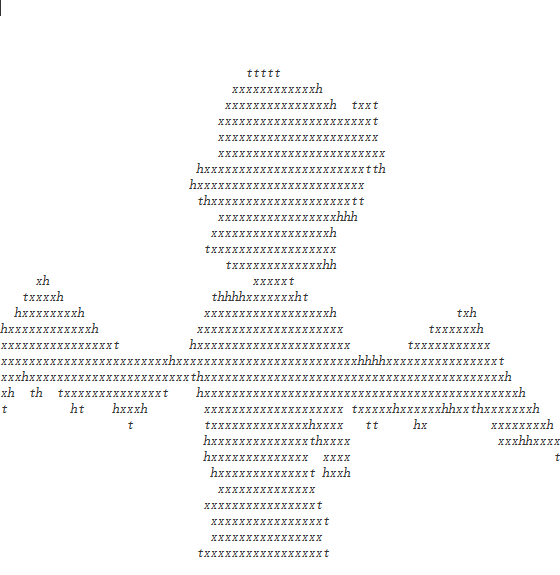（多符号输出的字符画）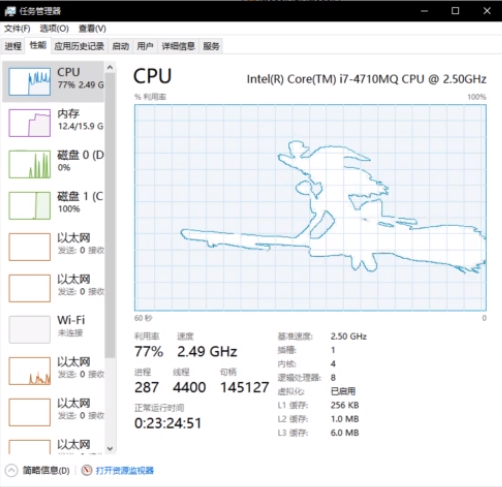# 0x00 准备阶段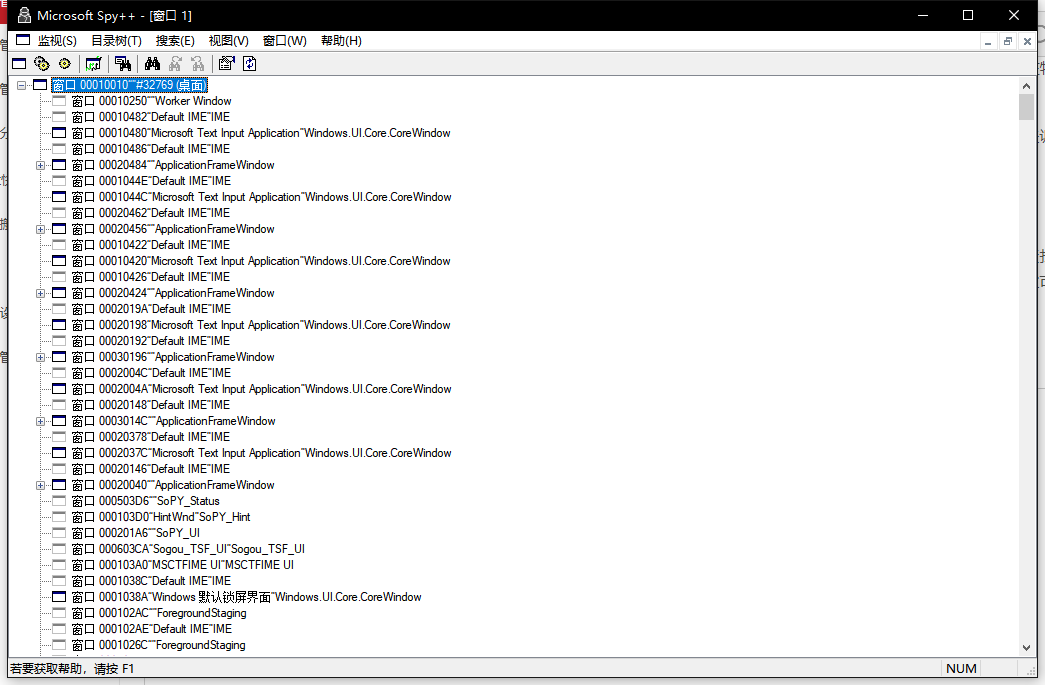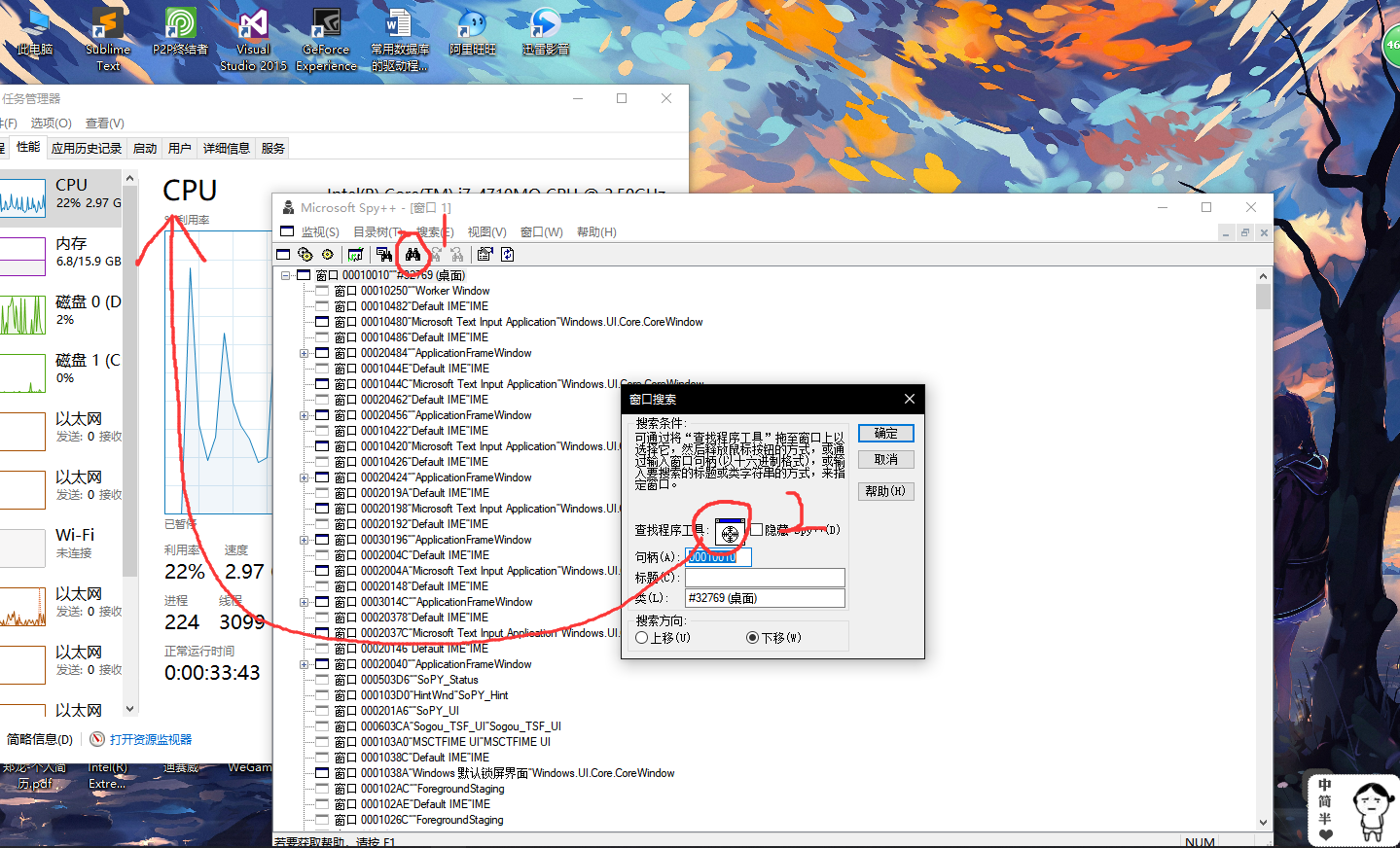点击①这个小望远镜 ，在弹出的小窗口中，拖动②中的指针到任务管理器窗口上，然后松手。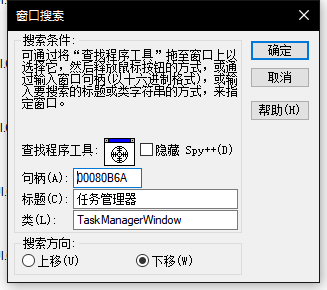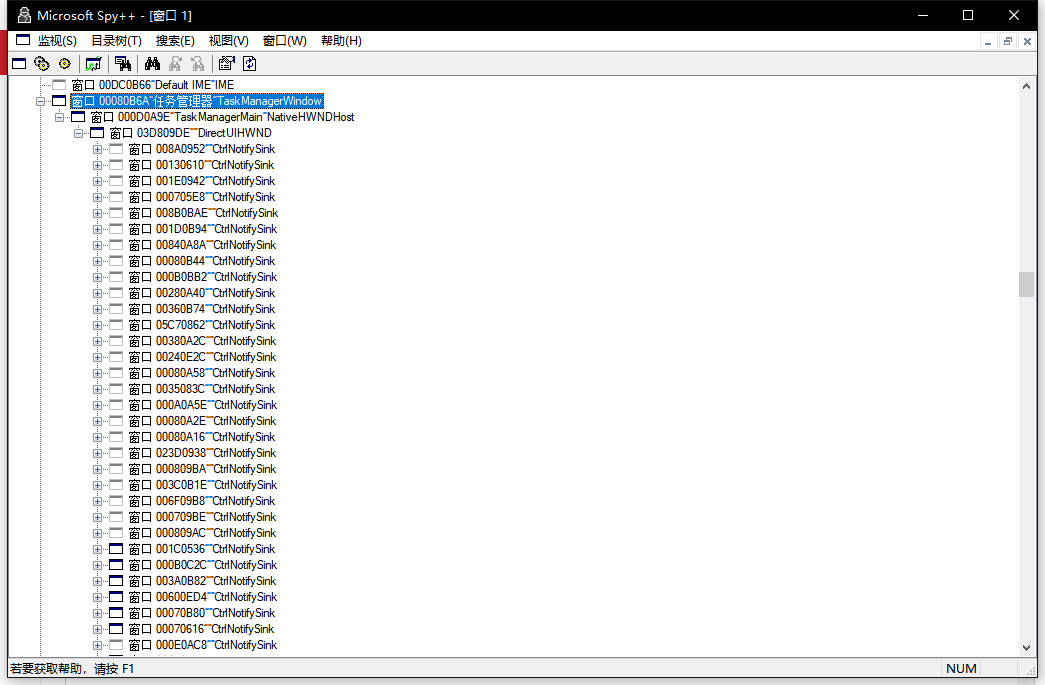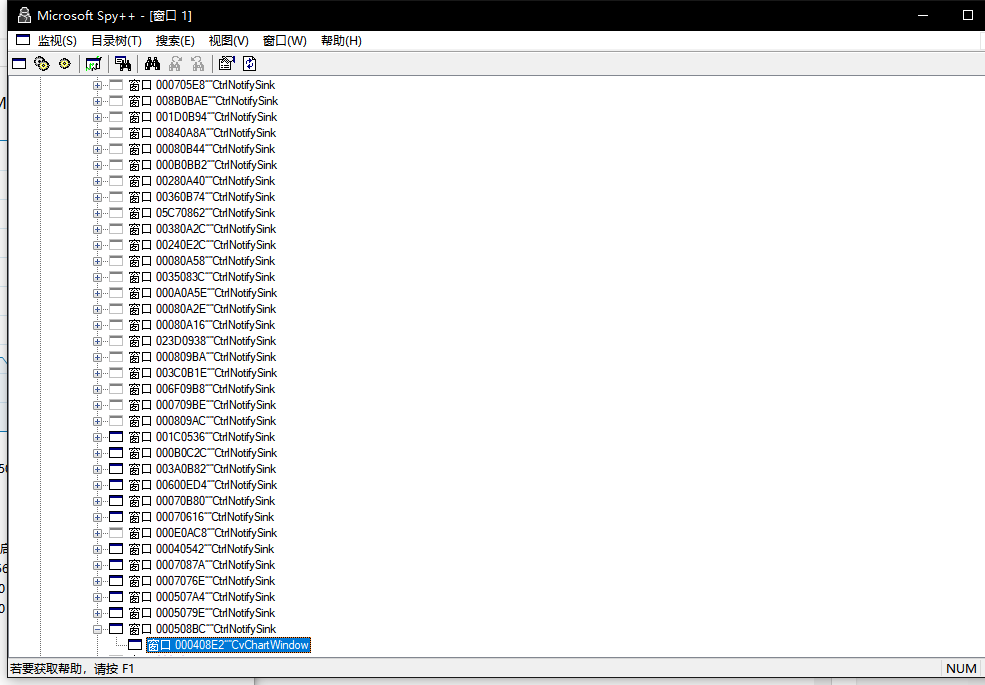Spy++也很快搜索了出来。

# 0x01 使用代码获取目标句柄

FindWindow函数原型是这样的：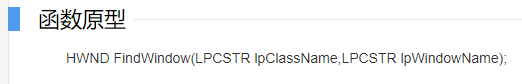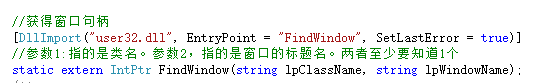在C#中使用IntPtr保存目标句柄。函数使用方式如下：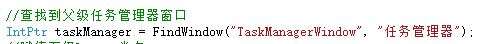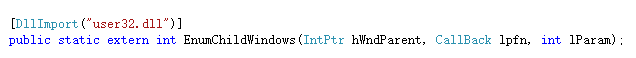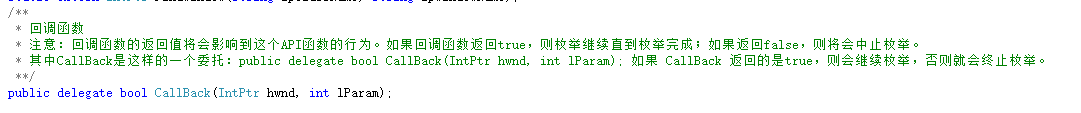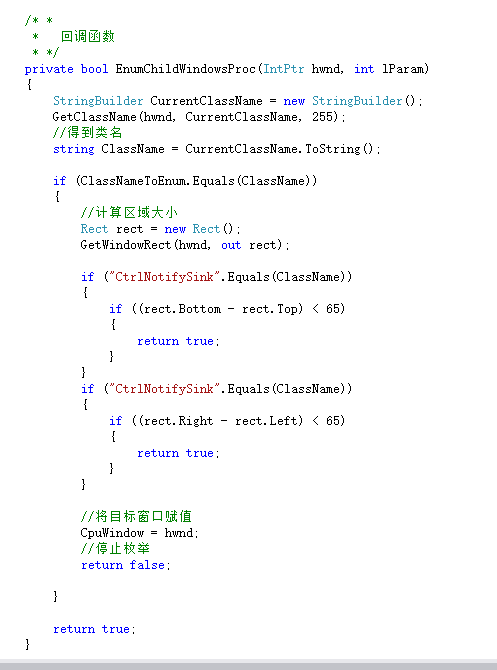实例化并使用：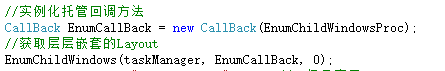# 0x02 在目标区域进行绘图

DrawImage需要的Image对象我们可以使用IO直接从文件中读取，代码实现如下：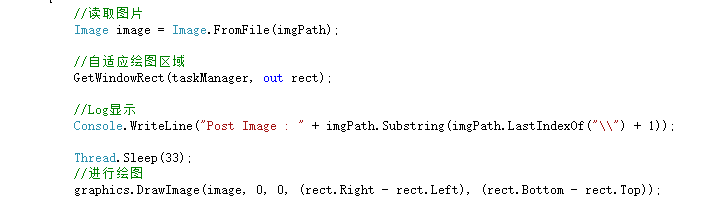# 0x03 对图像进行二值化处理

        /// <summary>
/// 求图像的灰度值
/// </summary>
/// <param name="color"></param>
/// <returns></returns>
private static int greyVal(Color color)
{
//int grey = (int)(color.R + color.G + color.B) / 3;//平均值法求灰度值
int grey = (int)(0.299 * color.R + 0.587 * color.G + 0.114 * color.B);//微调
return grey;
}

        /// <summary>
/// 二值化输出图像
/// </summary>
/// <param name="img"></param>
/// <param name="ft"></param>
public static Bitmap BinaryzationImage(Bitmap img, FrameTimer ft)
{
int w = mRect.Right - mRect.Left;
int h = mRect.Bottom - mRect.Top;

int UpPixel = 0;

for (int y = 0; y < img.Height; y++)   //把图像二值化
{
for (int x = 0; x < img.Width; x++)
{

int grayVal = greyVal(img.GetPixel(x, y)) > 192 ? 255 : 0;
if (grayVal < 128)
{
img.SetPixel(x, y, Color.FromArgb(grayVal, grayVal, grayVal));
}
}
}

for (int x = 0; x < img.Width; x++)   //边缘检测
{
for (int y = 0; y < img.Height; y++)
{

int grayVal = greyVal(img.GetPixel(x, y)) > 192 ? 255 : 0;
if (Math.Abs(grayVal - UpPixel) > 250)
{
img.SetPixel(x, y, Color.FromArgb(17, 152, 187));
}
UpPixel = grayVal;
}
}

for (int y = 0; y < img.Height; y++)   //边缘检测
{
for (int x = 0; x < img.Width; x++)
{

int grayVal = greyVal(img.GetPixel(x, y)) > 192 ? 255 : 0;
if (Math.Abs(grayVal - UpPixel) > 250)
{
img.SetPixel(x, y, Color.FromArgb(17, 152, 187));
}
if ((x == 0 || x == img.Width - 1) || (y == 0 || y == img.Height - 1))  //深蓝色边框
{
img.SetPixel(x, y, Color.FromArgb(17, 125, 187));
}
UpPixel = grayVal;
}
}

for (int y = 0; y < img.Height; y++)  //背景处理
{
for (int x = 0; x < img.Width; x++)
{

int grayVal = greyVal(img.GetPixel(x, y));
if (grayVal < 128)       //背景设为浅蓝色
{
img.SetPixel(x, y, Color.FromArgb(241, 246, 250));
}
if ((y % (h / 10) == 0) && y != 0)   //画方格的横线
{
img.SetPixel(x, y, Color.FromArgb(206, 226, 240));
}
if ((x % (w / 20) == (ft.tick() / 1000) * ((w / 40)) % (w / 20)) && x != 0)   //画方格的竖线
{
int rx = x;
img.SetPixel(rx, y, Color.FromArgb(206, 226, 240));

}
}
}

return img;

}

FrameTimer是一个自定义的计算时间的类。因为为了模拟任务管理器的动作，所以还需要在原图上画出横纵的表格线。而在FrameTimer中通过tick()方法，则可以判断当前播放到了第几秒，从而在相应的位置画出图像。

FrameTimer类定义如下：

    /// <summary>
/// 帧动画定时器类
/// </summary>
class FrameTimer
{
/// <summary>
/// 开始时间
/// </summary>
public DateTime startTime;

public FrameTimer()
{
//设置开始时间
this.startTime = DateTime.Now;
}

public int tick()
{
DateTime now = DateTime.Now;

//计算差值
TimeSpan ts = now - this.startTime;
int differenceValue = ts.Seconds;

//返回差值
return differenceValue;
}

};

# 0x04 实际操作中遇到的一点小坑

1. 将Bitmap图转换为byte[]数组，在内存中直接对byte[]数组进行操作，操作完成后再将byte[]数组转换成Bitmap输出，这样就不再需要不停地GetPixel和SetPixel了。
2. 使用指针遍历像素点，直接对像素点进行修改操作。# 0x05 测试效果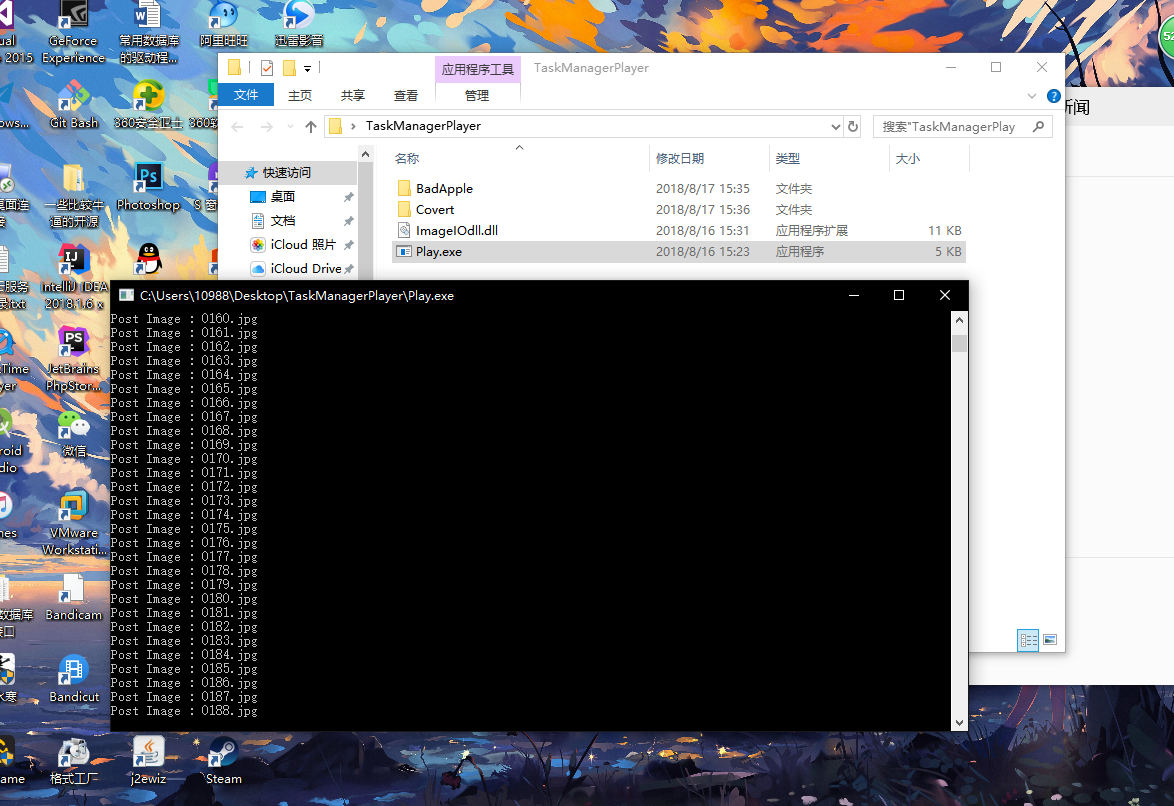BadApple是原始资源文件夹，Covert是二值化之后的资源文件。这里需要保证“BadApple”和“Covert”不被删除，否则如果删除了前者，则会抛出文件读取异常，删除后者你可能就要等程序二值化等好久啦！# 0x06 发布、测试及修改09-1389

#### 让任务管理器播放动画05-21850

09-14878

#### 使用WINDOWS任务管理器绘制正弦曲线

03-236684

12-0541

11-21

01-075910

06-22

12-182661

12-23

10-301万+

#### 哔哩哔哩怎么下载视频?不会我教你

03-085857

#### Unity的动画重新播放/暂停/继续播放（Animation/Animator）©️2020 CSDN 皮肤主题: 数字20 设计师: CSDN官方博客点击重新获取扫码支付1.余额是钱包充值的虚拟货币，按照1:1的比例进行支付金额的抵扣。
2.余额无法直接购买下载，可以购买VIP、C币套餐、付费专栏及课程。余额充值